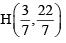Courses

# Competition Level Test: Straight Line- 3

## 30 Questions MCQ Test Mathematics For JEE | Competition Level Test: Straight Line- 3

Description
This mock test of Competition Level Test: Straight Line- 3 for JEE helps you for every JEE entrance exam. This contains 30 Multiple Choice Questions for JEE Competition Level Test: Straight Line- 3 (mcq) to study with solutions a complete question bank. The solved questions answers in this Competition Level Test: Straight Line- 3 quiz give you a good mix of easy questions and tough questions. JEE students definitely take this Competition Level Test: Straight Line- 3 exercise for a better result in the exam. You can find other Competition Level Test: Straight Line- 3 extra questions, long questions & short questions for JEE on EduRev as well by searching above.
QUESTION: 1

### Consider a family of straight lines (x + y) + λ(2x - y + 1) = 0 . Find the equation of the straight line belonging to this family that is farthest from (1,-3)

Solution:

AT FIRST FIND THE COMMON PONT OF THIS FAMILY WHICH IS A (-1/3 , 1/3)
THEN FOR THE LINE TO BE AT THE FARTHEST DISTANCE FROM B(1,-3) THE LINE SHOULD BE PREPENDICULAR TO AB THEREFORE THE SLOPE OF LINE m= 2/5
EQUATION OF LINE IS 2x + 2/3 = 5y - 5/3 WHICH IS HENCE 6x-15y-7=0

QUESTION: 2

### One side of an equilateral triangle is 3x+4y=7 and its vertex is (1,2). Then the length of the side of the triangle is

Solution: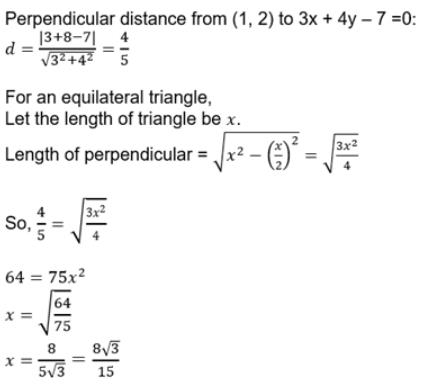QUESTION: 3

### Find the equation of the bisector of the angle between the lines x+2y–11 = 0, 3x–6y–5 = 0 which contains the point (1,–3)

Solution:

x+2y−11=0    ..(1)

3x−6y−5=0    ..(2)

Point of intersection of (1) and (2)

x=19/3

y=7/3

Equation passing through (19/3,7/3) and (1,-3)

y-7/3=1616(x-19/3)

x-y-4=0

QUESTION: 4

A straight line which make equal intercepts on +ve x and y axes and which is at a distance '1' unit from the origin intersects the straight line y = 2x + 3 + √2 at (x0, y0) then 2x0 + y0

Solution:

Equation of the straight line having equal intercepts is x + y = k and proceed.

QUESTION: 5

The locus of the image of the point (2, 3) in the line (x -2y +3) + λ (2x -3y + 4) = 0 (λ∈R) is

Solution:

A(2,3),B(1,2),P(x,y)
AB = BP

QUESTION: 6

A straight line L with negative slope passes through the point (8, 2) and cuts the positive coordinate axes at points P and Q. As L varies, the absolute minimum value of OP+OQ is (O is origin)

Solution:

The equation of the line L be x coordinates of P and Q are 3x + 4y = 9 and y = mx + 1 and P (-3, 4) .
So Q (0, 1)
So, absolute minimum value of x

QUESTION: 7

The number of integral values of m for which the x coordinate of the point of intersection of the lines 3x + 4y = 9 and y = mx + 1 is also an integer is

Solution:

Solving given equations we get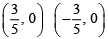is an integer, if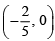(-2, 0) y = mx So m has two integral values.

QUESTION: 8

Area of the parallelogram formed by the lines y = mx, y = mx+ 1, y = nx and y = nx+ 1 equals (m ≠ n)

Solution:

If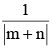be the distance between parallel sides and θ be the angle between adjacent sides, then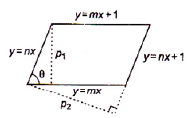Requried area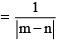Whre  y = mx + 1, x (distance between | | lines) and π/4 (0,3)

QUESTION: 9

If the midpoint of sides of a triangle are (a, 0), (0, b) and (-a, -b), then the centroid of the triangle is

Solution:
QUESTION: 10

The triangle ABC has medians AD, BE, CF. AD lies along the line y = x + 3, BE lies along the line y = 2x + 4, AB has length 60 and angle C = 90°, then the area of ΔABC is

Solution:
QUESTION: 11

Let A(1, 0), B(0, –2) and P(a, b) be a third point that lies on the straight line 'x - y= 0' such that, |PA + PB| is minimum then the values of |a + b| =

Solution:
QUESTION: 12

Let A(0, 0), B(3, 0), C(2, 1) then area of the square inscribed in the triangle such that, one side of the square is taken along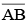Solution:
QUESTION: 13

Let O(0, 0), A(–1, 1) the point ‘A’ is rotated 60o in anticlockwise sense about origin then the slope of line joining original and the new positions of ‘A’ is

Solution:
QUESTION: 14

Let y = 2x + c1, y = 2x + c2 meet X-axis at A1, A2 respectively and Y-axis at B1, B2 respectively then the locus of the point of intersection of lines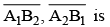Solution: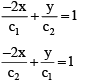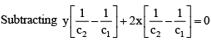QUESTION: 15

Let A(2, 3) and B is the image of A with respect to the lines (2x - 3y + 4) + k (x - 2y + 3) = 0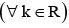then the locus of B is

Solution:

The given line is
(2x - 3y + 4) + k (x - 2y + 3) = 0
as x−2y=−3
2x−3y=−4
multiplying eqn(2) by 2 and subtracting we get, y=2
so, x=−3+4=1
so point of intersection P is (1,2)
A′ is the reflection of A
as, AP=BP=A′P
so, √(2-1)2+(3-2)2] =[(h-1)2+(k-2)2]1/2
1+1=h2+k2-2h-4k+1+4
h2+k2−2h−4k+3=0
x2+y2-2x-4y+3=0

QUESTION: 16

Let 'P' be a point inside the equilateral triangle ABC, PA = 3, PB = 4, PC = 5 then the area of the triangle ABC (in square units)

Solution: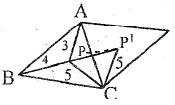Rotate the triangle by 60o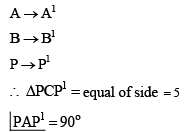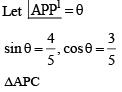AC2 = AP2 + PC2 - 2AP.PCcos(θ + 60°)

QUESTION: 17

Let a straight line passing through P(1, 4) with negative slope cuts the coordinate axes at A, B then the area of the triangle OAB when OA + OB is minimum is

Solution:

OA + OB = 1 + 4cot θ + 4 + tan θ
= 5 + tan θ + 4 cot θ
Attains minima only if tan θ = 4 cot θ

QUESTION: 18

The image of the curve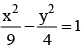with respect to the straight line x + y= 1 is

Solution:

Replace x by 1 – Y
y by 1 – X

QUESTION: 19

Let (a, b) lies on obtuse angle bisector of x + 2y + 1 = 0, 2x + y + 1 = 0 then the least value of (a - 1)2 + (b - 2)2 is

Solution:

Find short dist. From (1, 2)

QUESTION: 20

Let A(0, 0) and x + y - 1 = 0, 2x + 1 = 0 are the altitude, median from the vertices B, C respectively of ΔABC then the area of the triangle

Solution:
QUESTION: 21

Let (x - 1)2 + (y - 2)2 = 1/4 then the minimum value of " x + 3y+ 4"

Solution:
QUESTION: 22

Two vertical poles 20 mts, and 80 mts stand apart on a horizontal plane. The height of the point of intersection of the lines joining the top of each pole to the foot of others (in metres)

Solution:

The height "a" of the point E of intersection of the lines AD & BC drawn from the tops B & D of two poles AB & CD , to the feet of the other pole is given by LADDER THEOREM .
1/a = 1/AB + 1/CD
1/a = 1/20 + 1/80
= 5/80 = 1/16
So. a = 16 m

QUESTION: 23

A(3, 1), B(13, 6), C(13, 21), D(3, 16) forms a parallelogram, then the y-intercept of the line passing through (1, 0) and dividing the parallelogram into two equal areas

Solution:
QUESTION: 24

If the straight lines ax + by + p = 0 and x cosa + y sina = p are inclined at an angle p/4 and concurrent with the straight line x sina – y cosa = 0, then the value of a2 +b2 is

Solution:

ax+by+p=0   ...(1)
⇒by=−ax+p
⇒y=−a/bx+p/b
So, Slope,m1 = −a/b
and xcosθ+ysinθ−p=0    ..(2)
⇒ysinθ=−xcosθ+p
⇒y=−cosθ/sinθx+p/sinθ
So, Slope,m2=−cosθ/sinθ
Now, straight lines (1) and (2) include an angle π/4
i,e tanπ/4 = ∣∣(m1−m2)/(1+m1m2)∣∣
⇒ 1 = ∣∣(m1−m2)/(1+m1m2)∣∣
⇒1+m1m2|=|m1−m2|
⇒∣∣1+(−a/b×(−cosθ/sinθ))∣∣
=∣∣−a/b−(−cosθ/sinθ)∣∣
⇒∣∣(bsinθ+acosθ)/bsinθ∣∣
=∣∣(−asinθ+bcosθ)/bsinθ∣∣
⇒|bsinθ+acosθ|=|−asinθ+bcosθ|
Squaring both sides, we get
b2sin2θ+a2cos2θ+2absinθ cosθ=a2sin2θ+b2cos2θ−2absinθ cosθ    ...(3)
Given three lines are concurrent
Now, we will find the point of intersection of
xcosθ+ysinθ=p and xsinθ−ycosθ=0
which is x=pcosθ and y=psinθNow, point of intersection will satisfy (1)
i.e apcosθ+bpsinθ+p=0
⇒apcosθ+bpsinθ=−p
⇒acosθ+bsinθ=−1
Squaring both sides, we get
⇒a2cos2θ+b2sin2θ+2absinθ cosθ=1   ...(4)
Put the value of (4) in (3), we get
a2sin2θ+b2cos2θ−2absinθ cosθ =1   ....(5)
Adding (4) and (5), we get
a2cos2θ+b2sin2θ+2absinθ cosθ+a2sin2θ+b2cos2θ−2absinθ cosθ=1+1
⇒a2(sin2θ+cos2θ)+b2(sin2θ+cos2θ)=2
⇒a2+b2=2

QUESTION: 25

Let (1, 2), (3, 8), (4, 1) are three vertices of a parallelogram the sum of all possible x coordinates of the fourth vertex of the parallelogram is

Solution:

Let ABCD be a parallelogram.
then A (1,2), B (3,8) and C (4,1). Let the coordinates of D be (x,y)
Since the diagonals of a parallelogram bisect each other
Coordinates of the mid-point of AC = Coordinates of the mid-point of BD
(1+4)/2 , (2+1)/2 = (3+x)/2 , (8+y)/2
5/2 , 3/2     (3+x)/2 , (8+y)/2
2.5, 1.5       , (3+x)/2 , (8+y)/2
(3+x)/2 = 2.5 , 3/2 = (8+y)/2
(3+x) = 5 ,     -5 = y
2 = x        , -5 = y
∴ Coordinates of the fourth vertex are (2, -5)

QUESTION: 26

The orthocenter of the triangle formed by the lines x - 7y + 6 = 0, 2x - 5y - 6 = 0 and 7x + y - 8 = 0 is

Solution:

x-7y+6 = 0
m1 = -1/-7   = 1/7
7x+y-8 = 0
m2 = -7/1
Slope = 1/7 * (-7)
= 1
B is orthocentre of triangle ABC
x-7y = -6    ---------------------------(1)
7x+y = 8   ---------------------------(2)
y =8-7x    ----------------------------(3)
Put eq (3) in eq(1)
x-7(8-7x) = -6
x-56+49x = -6
50x = 50
x = 1
Similarly y = 1
Points are (1,1)

QUESTION: 27

The equations x = t3 + 9 and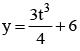represents a straight line where t is a parameter. Then y-intercept of the line is :

Solution:

x=0
Put into x= t3 + 9
0 = t3 + 9
t3 = -9
y = 3(t3)/4 + 6
y = 3(-9)/4 + 6
y= -¾

QUESTION: 28

The vertices of triangle ABC are A(–1,–7), B(5, 1) and C(1, 4). The equation of the bisector of the angle ABC of ΔABC is :

Solution: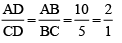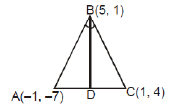QUESTION: 29

y - 1 = m1 x - 3 and y - 3 = m2 (x - 1) are two family straight lines (m1, m2R), at right angles to each other. The locus of their point of intersection is :

Solution: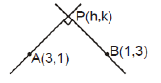Locus of P (h, k) is circle with AB as diameter
(x -3)(x -1) + (y -1)(y -3) = 0

QUESTION: 30

The equation of the line passing through the intersection of the lines 3x + 4y = -5, 4x + 6y = 6 and perpendicular to 7x - 5y + 3 = 0 is :

Solution: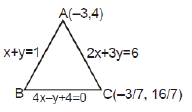Equation of altitude on BC
x + 4y= 13
Equation of altitude on AB
7x - 7y + 19 = 0
⇒